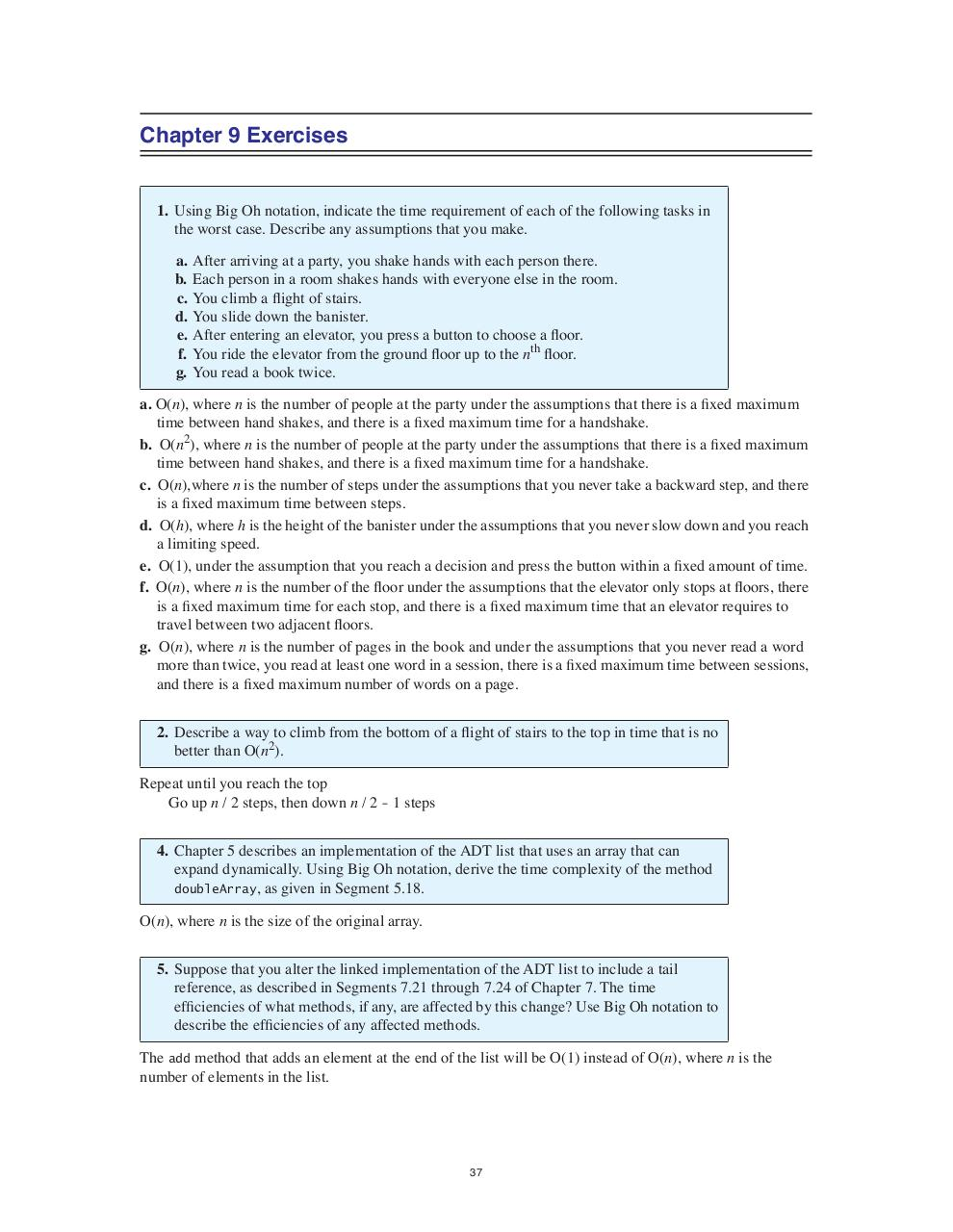# 49145 Carrano ExerSol2e (1).37 .pdf

### File information

Original filename: 49145-Carrano_ExerSol2e (1).37.pdf

This PDF 1.4 document has been generated by / iTextÂ® 5.4.5 Â©2000-2013 1T3XT BVBA (ONLINE PDF SERVICES; licensed version), and has been sent on pdf-archive.com on 16/02/2014 at 03:22, from IP address 129.107.x.x. The current document download page has been viewed 1152 times.
File size: 98 KB (1 page).
Privacy: public file

49145-Carrano_ExerSol2e (1).37.pdf (PDF, 98 KB)

### Document preview

Chapter 9 Exercises

1. Using Big Oh notation, indicate the time requirement of each of the following tasks in
the worst case. Describe any assumptions that you make.
a.
b.
c.
d.
e.
f.
g.

After arriving at a party, you shake hands with each person there.
Each person in a room shakes hands with everyone else in the room.
You climb a flight of stairs.
You slide down the banister.
After entering an elevator, you press a button to choose a floor.
You ride the elevator from the ground floor up to the nth floor.

a. O(n), where n is the number of people at the party under the assumptions that there is a fixed maximum
time between hand shakes, and there is a fixed maximum time for a handshake.
b. O(n2), where n is the number of people at the party under the assumptions that there is a fixed maximum
time between hand shakes, and there is a fixed maximum time for a handshake.
c. O(n),where n is the number of steps under the assumptions that you never take a backward step, and there
is a fixed maximum time between steps.
d. O(h), where h is the height of the banister under the assumptions that you never slow down and you reach
a limiting speed.
e. O(1), under the assumption that you reach a decision and press the button within a fixed amount of time.
f. O(n), where n is the number of the floor under the assumptions that the elevator only stops at floors, there
is a fixed maximum time for each stop, and there is a fixed maximum time that an elevator requires to
g. O(n), where n is the number of pages in the book and under the assumptions that you never read a word
more than twice, you read at least one word in a session, there is a fixed maximum time between sessions,
and there is a fixed maximum number of words on a page.
2. Describe a way to climb from the bottom of a flight of stairs to the top in time that is no
better than O(n2).
Repeat until you reach the top
Go up n / 2 steps, then down n / 2 - 1 steps
4. Chapter 5 describes an implementation of the ADT list that uses an array that can
expand dynamically. Using Big Oh notation, derive the time complexity of the method
doubleArray, as given in Segment 5.18.
O(n), where n is the size of the original array.
5. Suppose that you alter the linked implementation of the ADT list to include a tail
reference, as described in Segments 7.21 through 7.24 of Chapter 7. The time
efficiencies of what methods, if any, are affected by this change? Use Big Oh notation to
describe the efficiencies of any affected methods.
The add method that adds an element at the end of the list will be O(1) instead of O(n), where n is the
number of elements in the list.

37#### HTML Code

Copy the following HTML code to share your document on a Website or Blog

#### QR Code### Related keywords